# NCERT Solutions for Class 10 Science Chapter 13

NCERT Solutions for Class 10 Science Chapter 13 – Magnetic Effects Of Electric Current, contains solutions to various questions in Exercises for Chapter 13. Magnetic Effects Of Electric Current Class 10 NCERT Solutions have been explained in a simple and easy to understand manner. We are providing all subjects which can be accessed by clicking here.

## Download NCERT Solutions for Class 10 Science Chapter 13 – Magnetic Effects Of Electric Current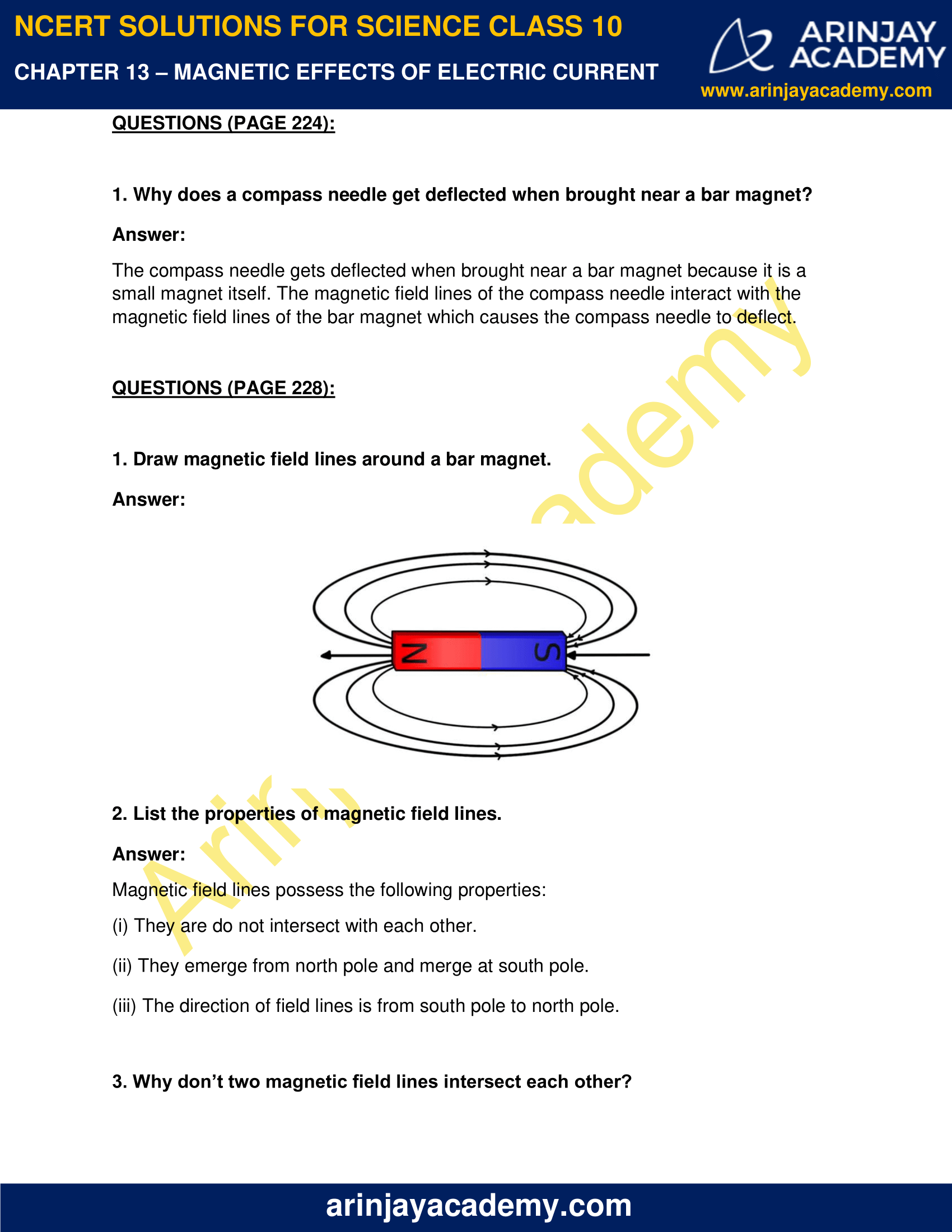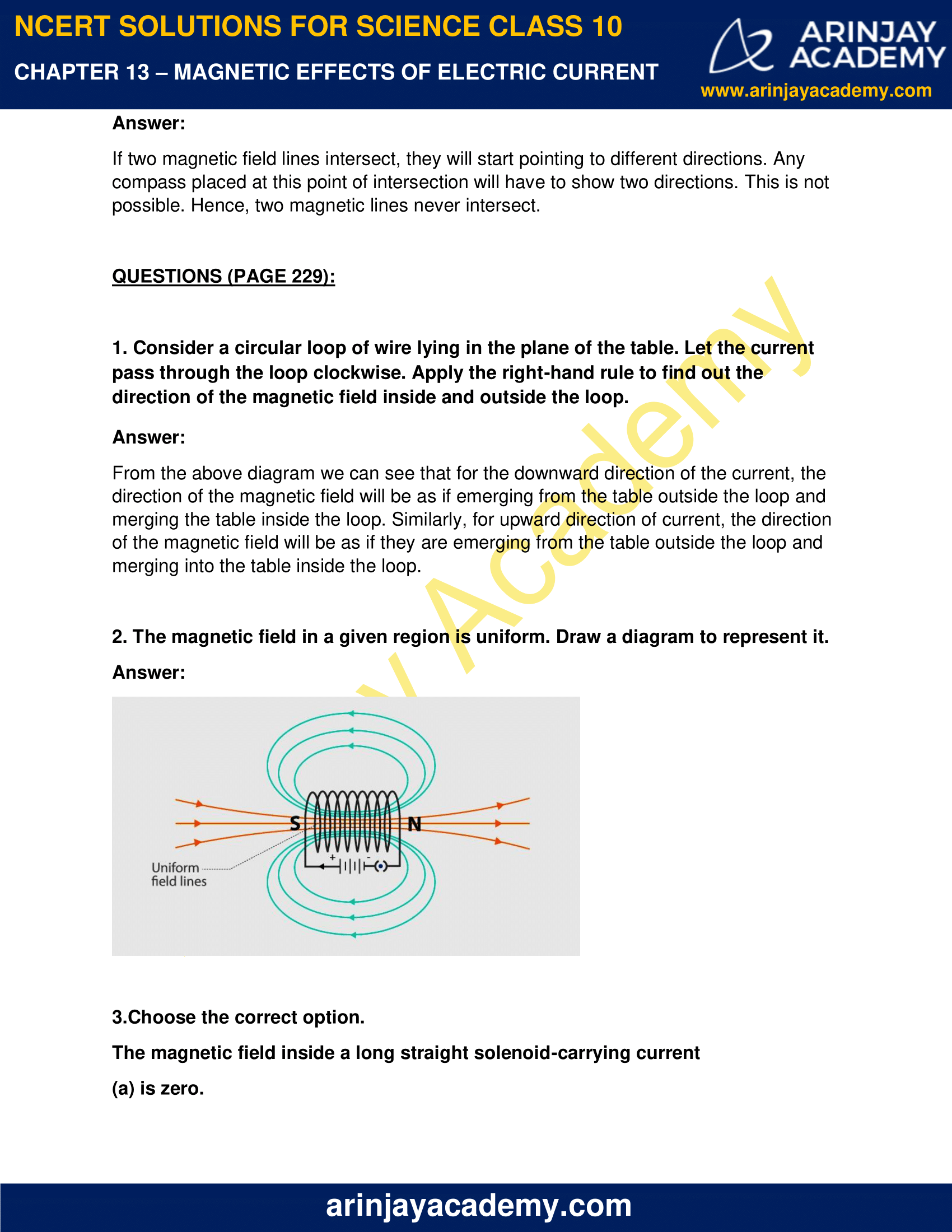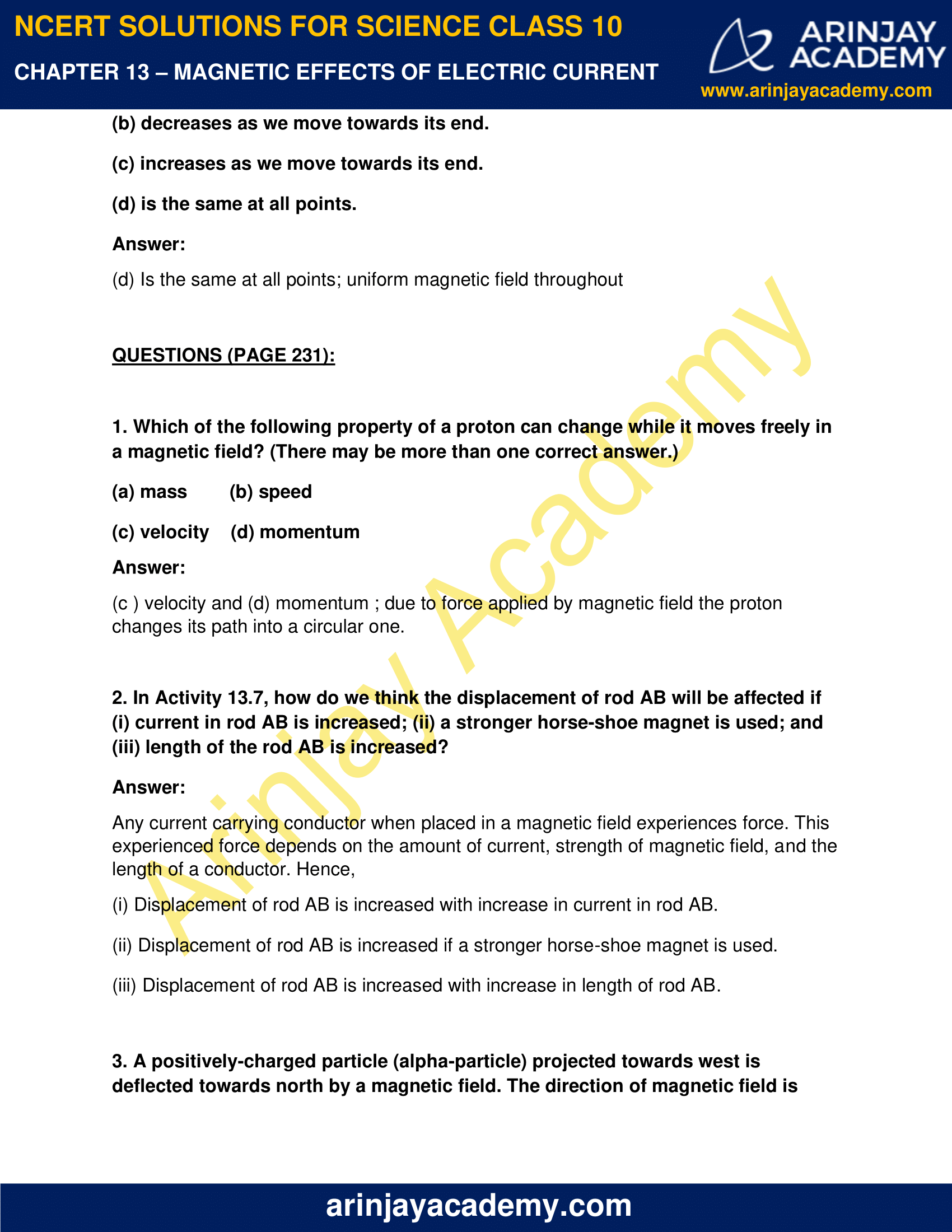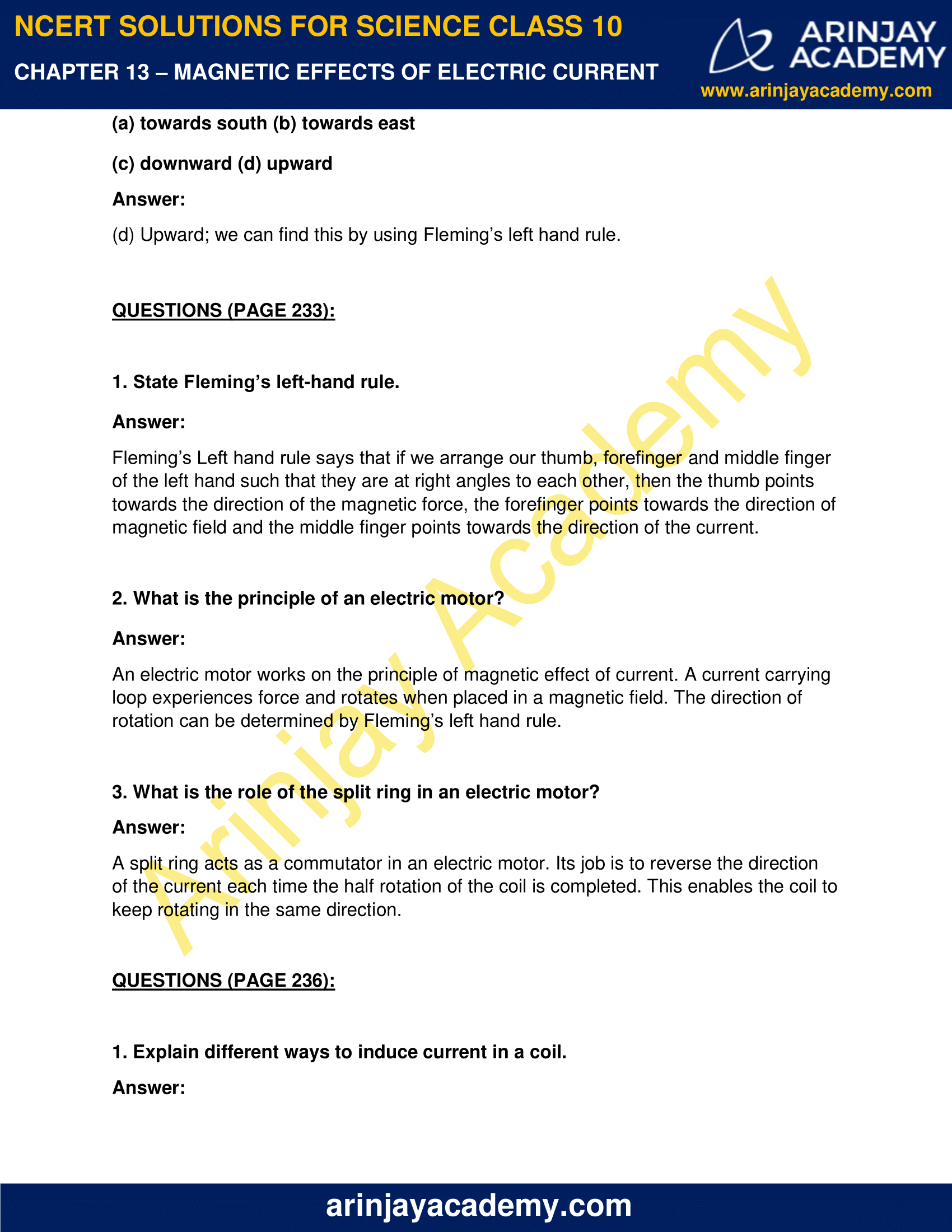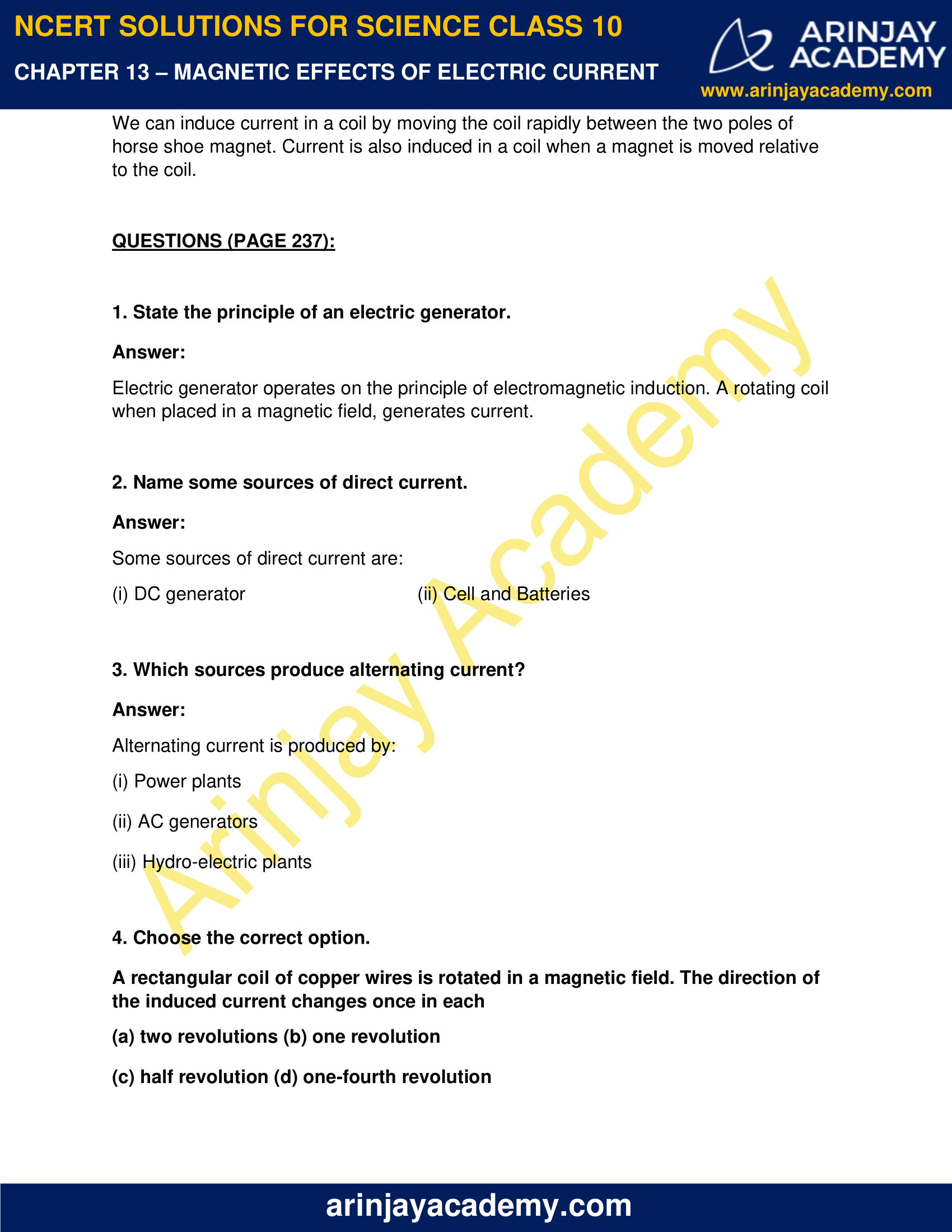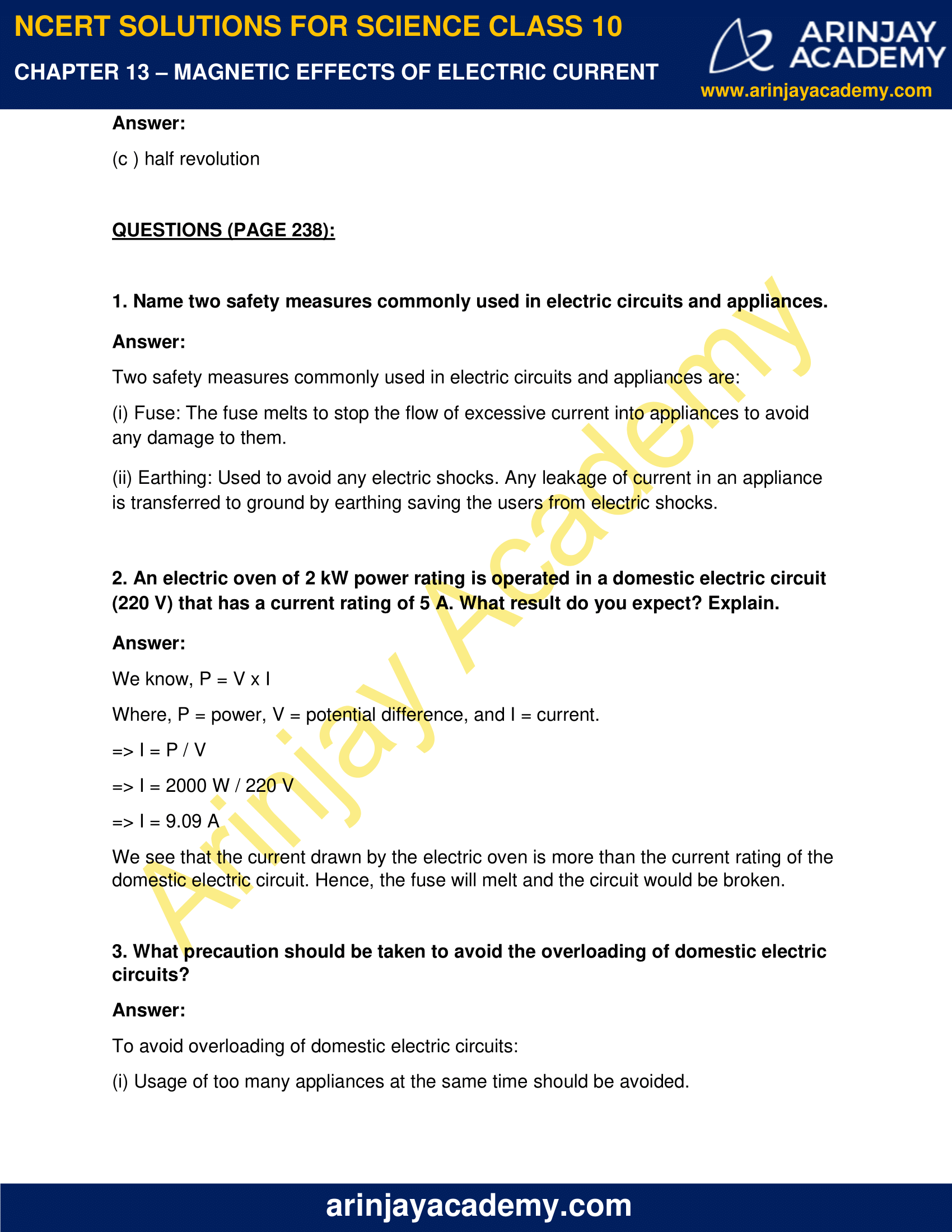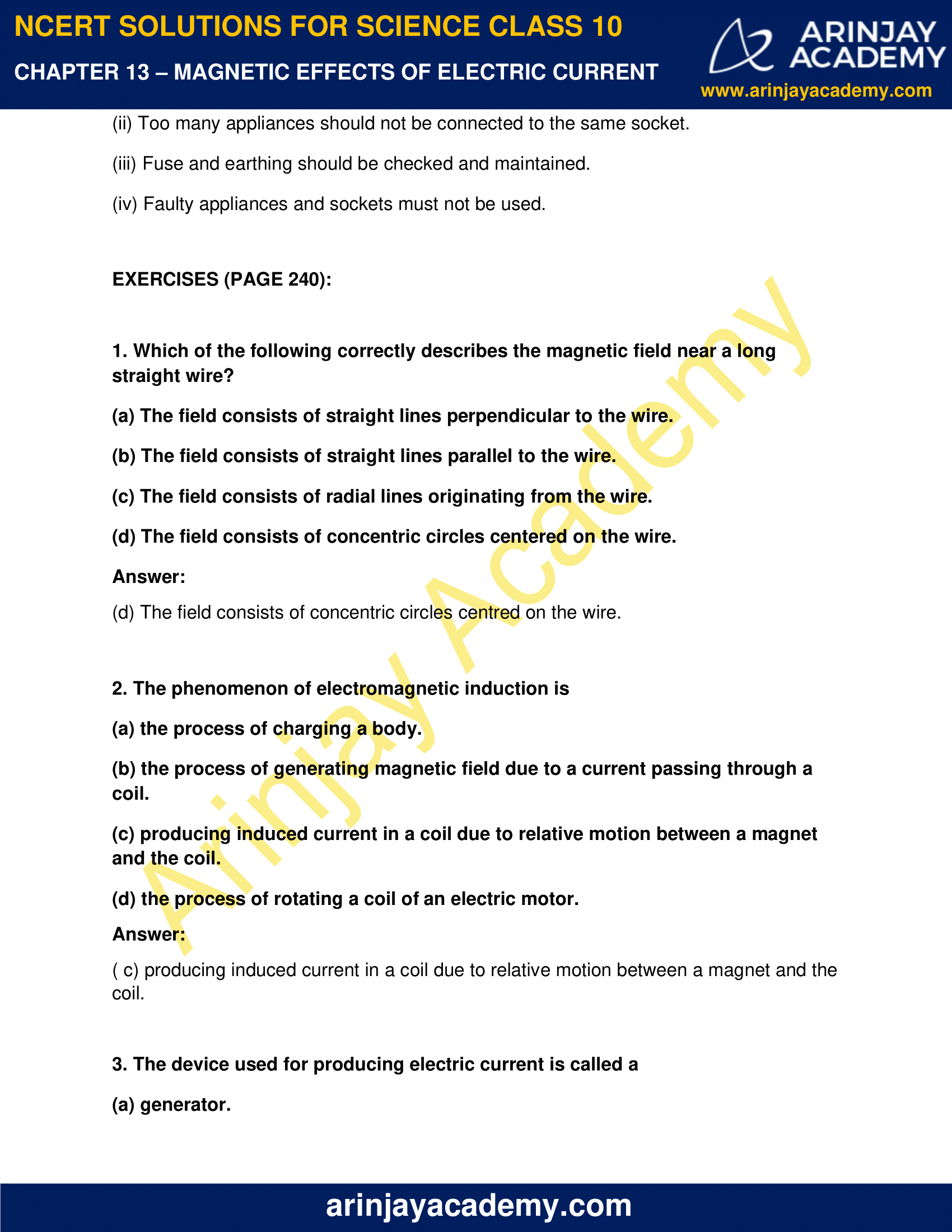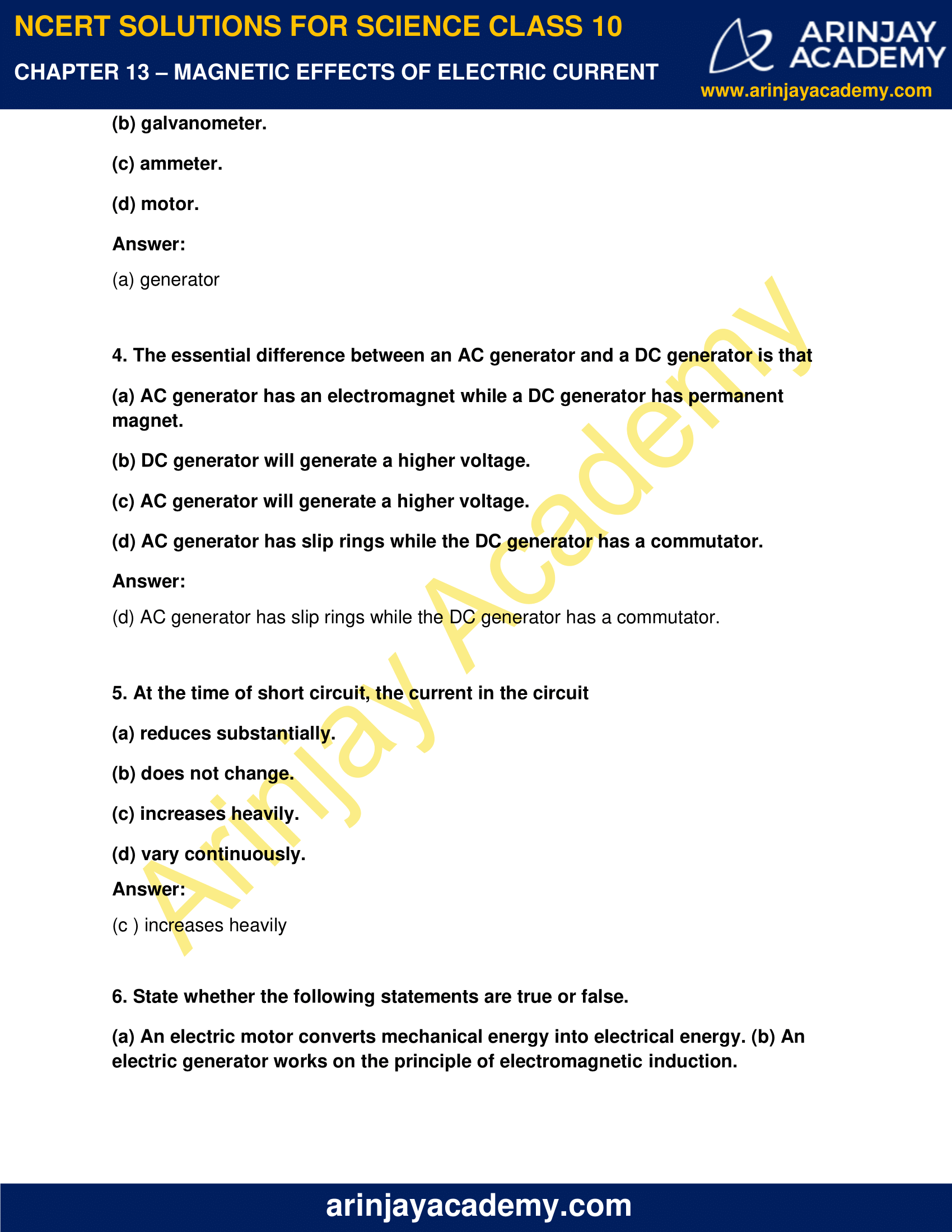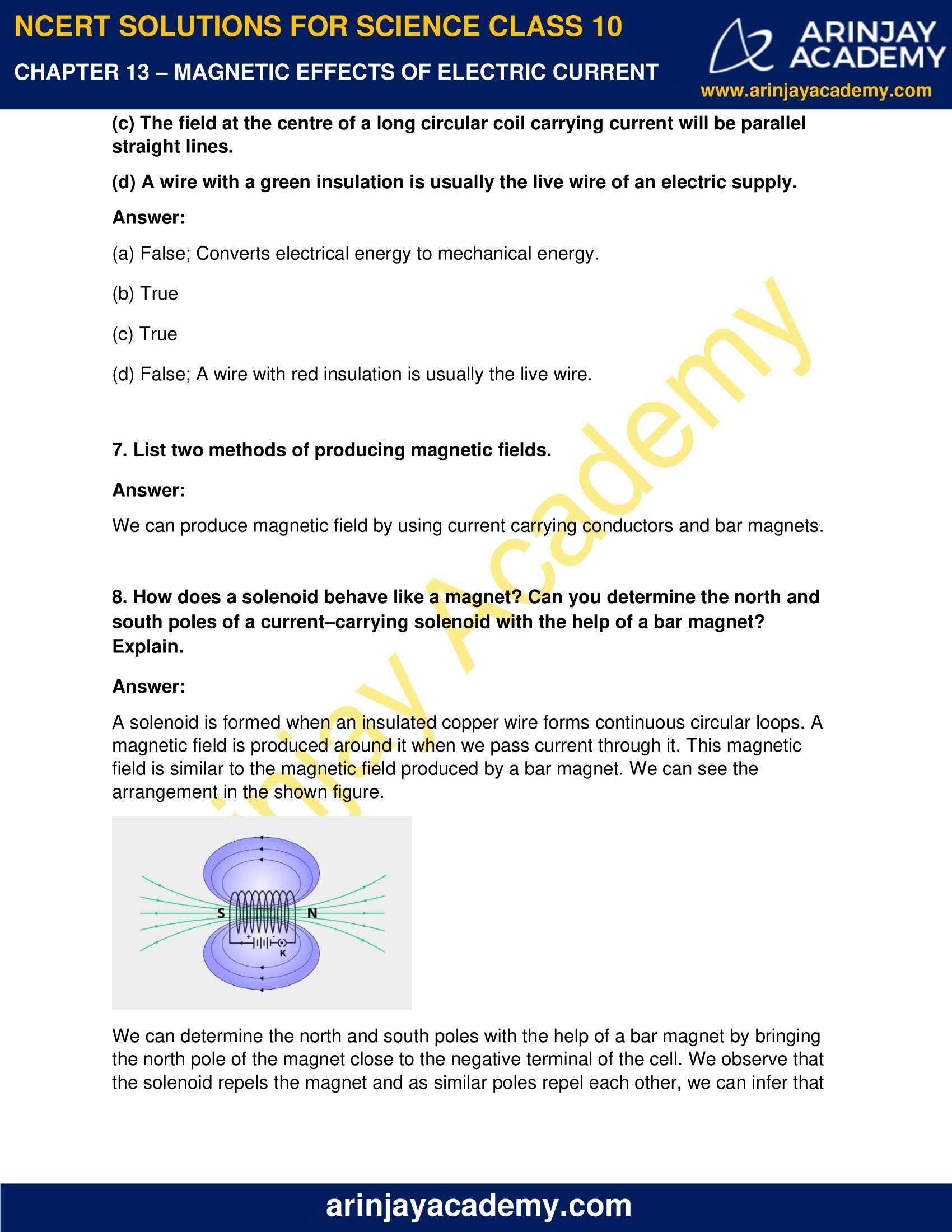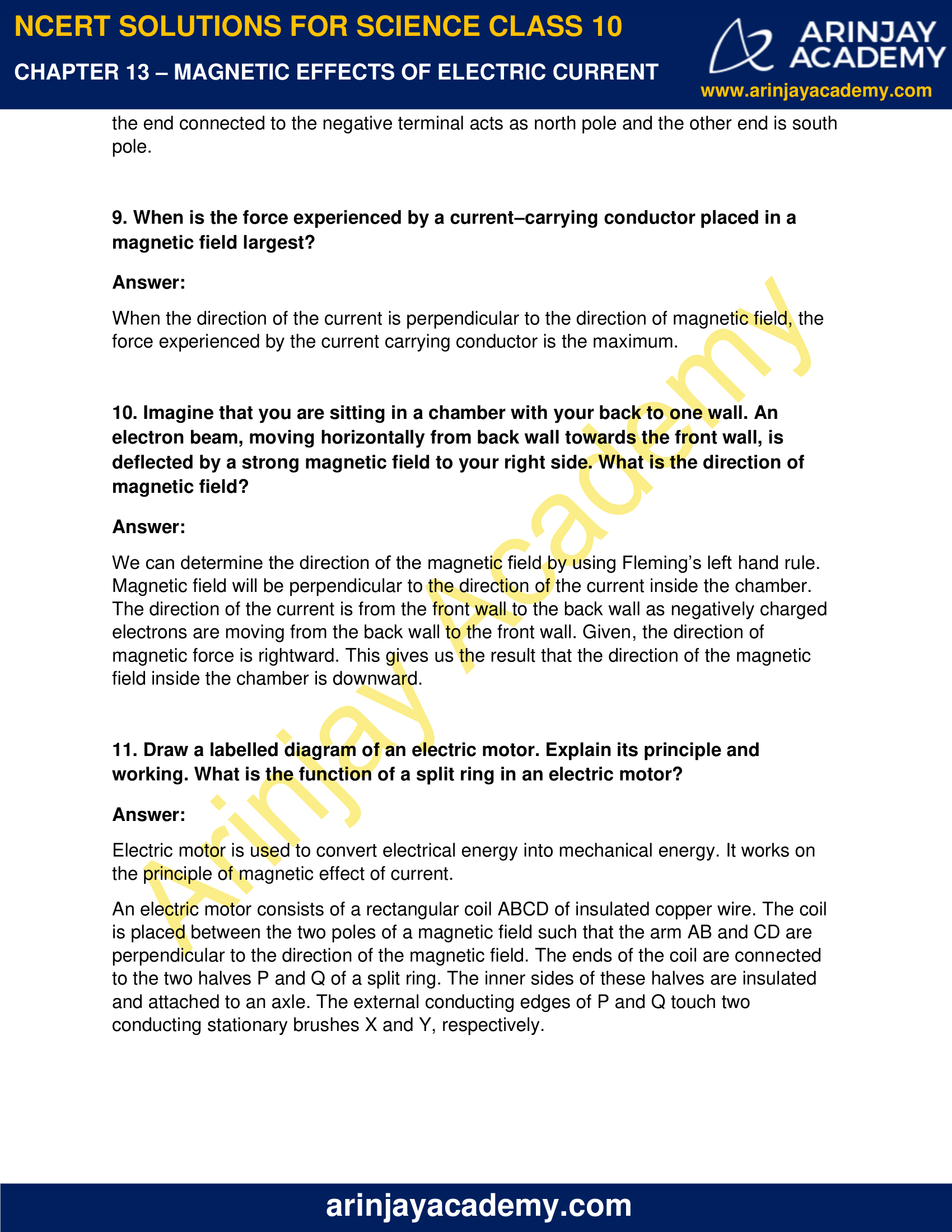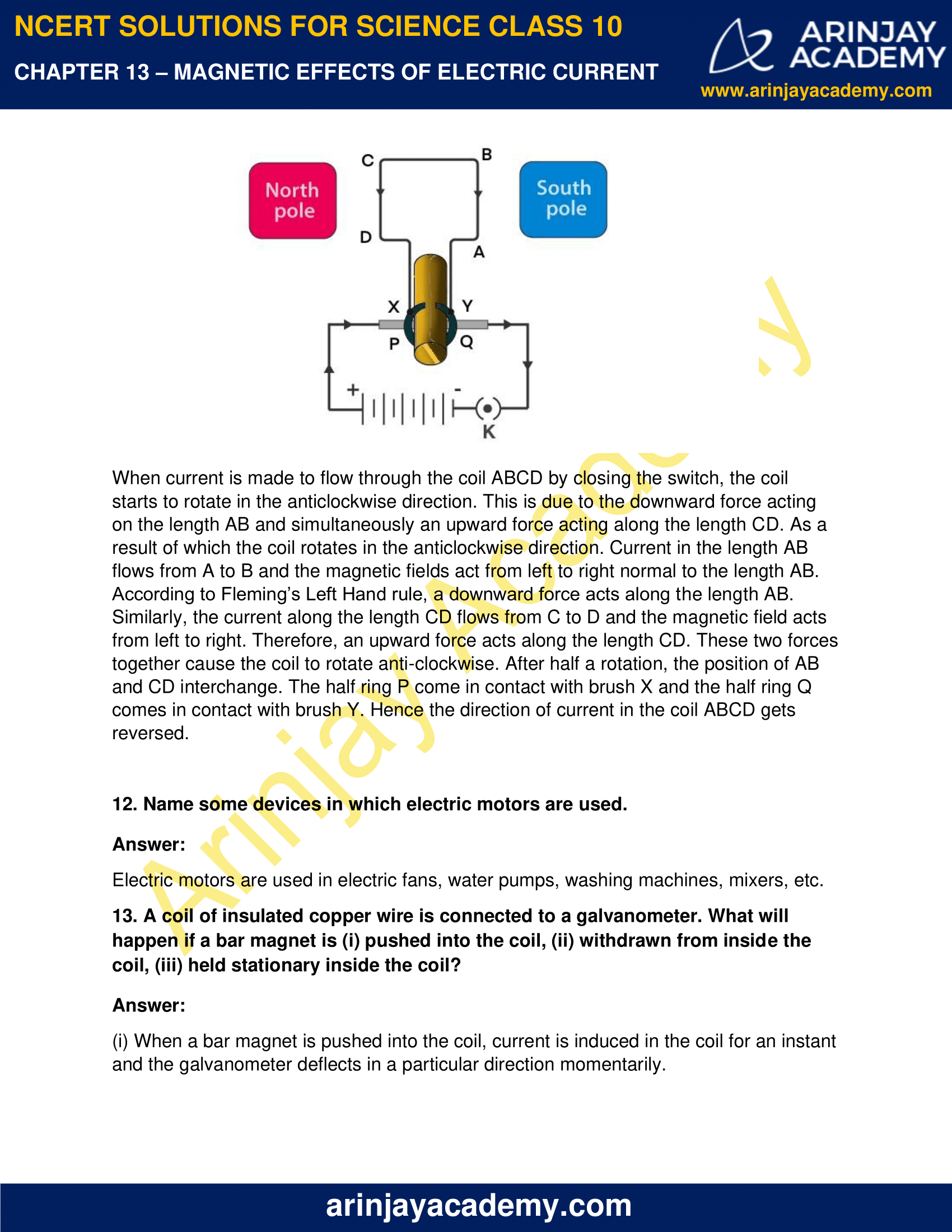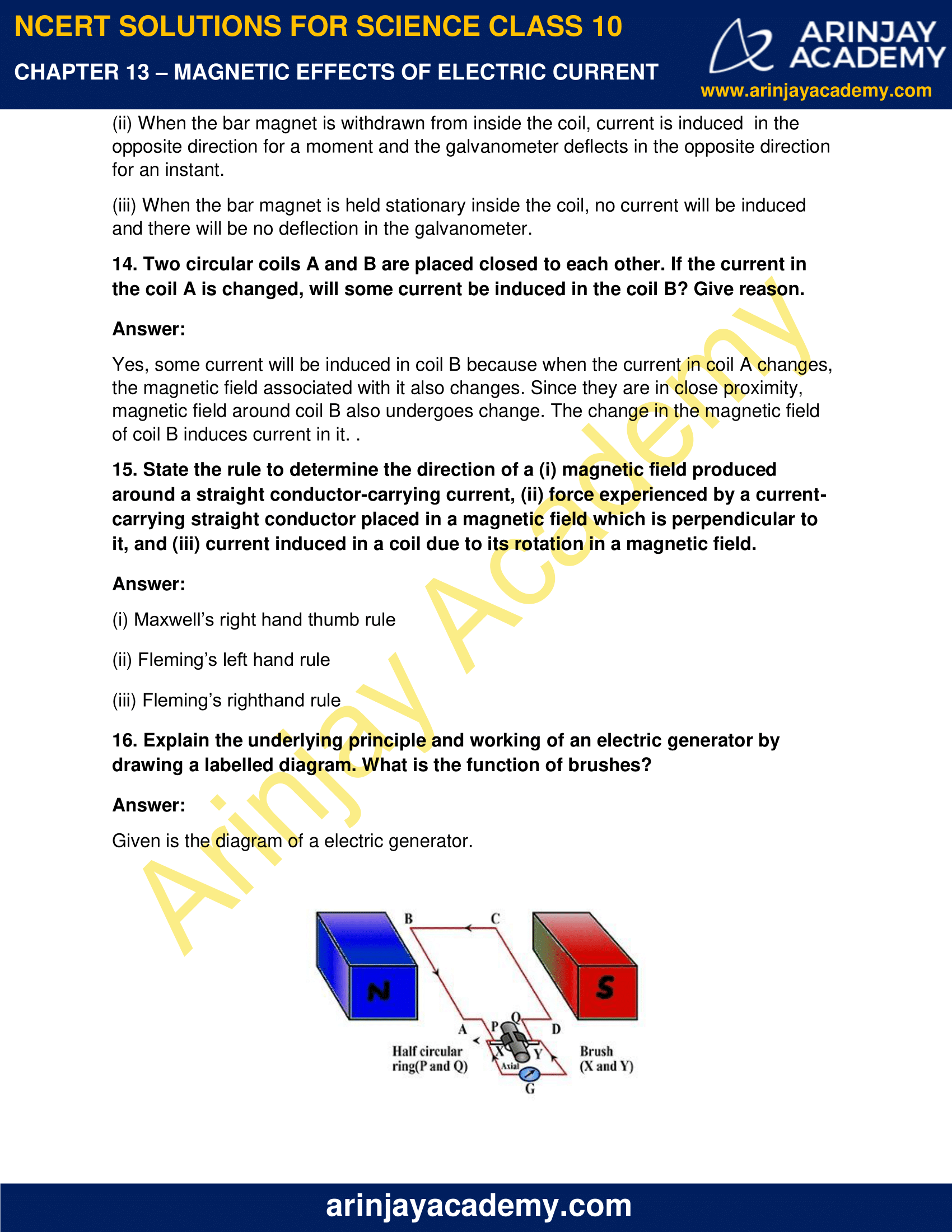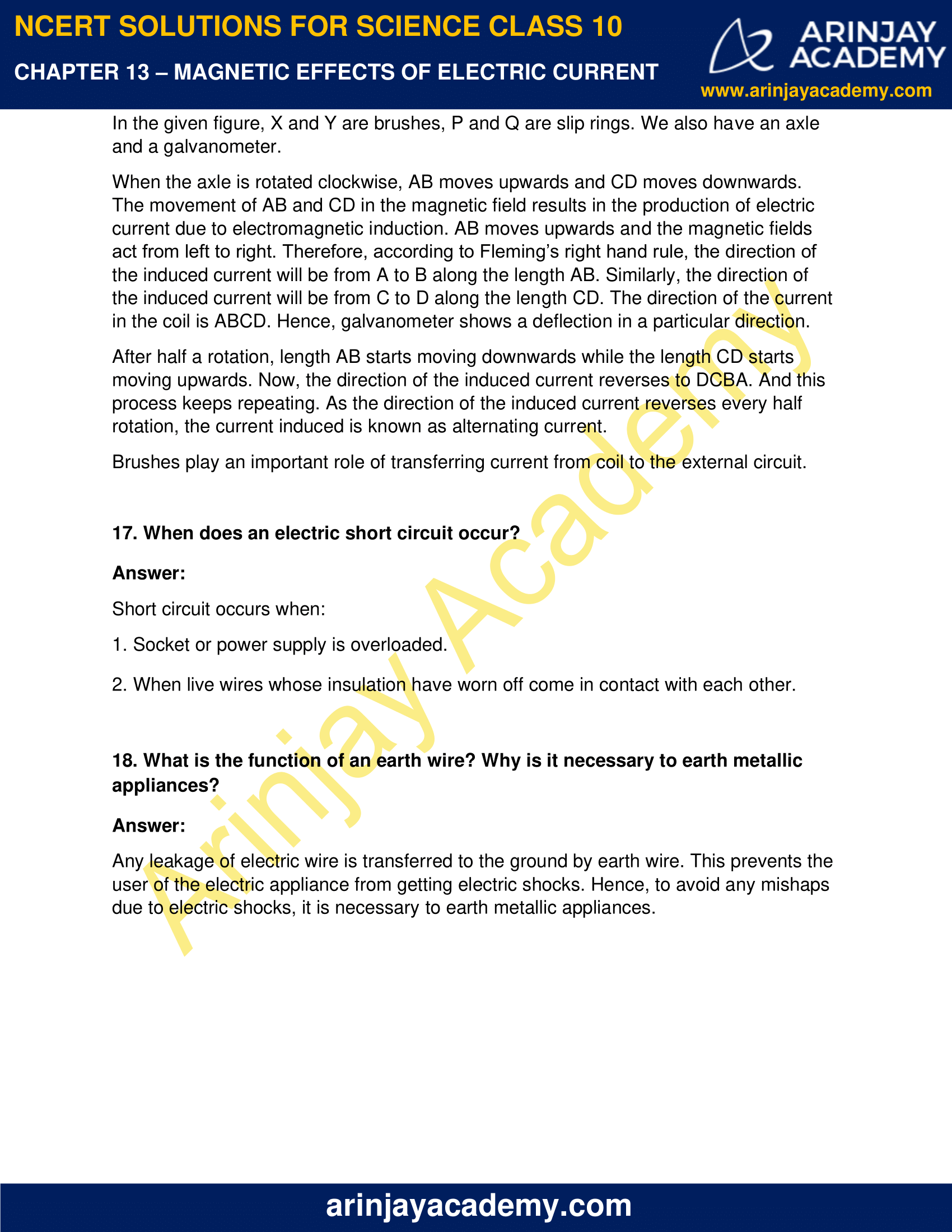### NCERT Solutions for Class 10 Science Chapter 13 – Magnetic Effects Of Electric Current – NCERT Exercises

NCERT Solutions for Class 10 Science Chapter 13 – Magnetic Effects Of Electric Current, Exercises includes all in text and exercise solved questions which helps you to understand the topic covered in Chapter 13 Magnetic Effects Of Electric Current Class 10, in a better manner to help you to score good marks in your examinations.

### NCERT Solutions for Class 10 Science Chapter 13 – Intext Questions – Page 224

1. Why does a compass needle get deflected when brought near a bar magnet?

The compass needle gets deflected when brought near a bar magnet because it is a small magnet itself. The magnetic field lines of the compass needle interact with the magnetic field lines of the bar magnet which causes the compass needle to deflect.

### Intext Questions – Page 228

1. Draw magnetic field lines around a bar magnet.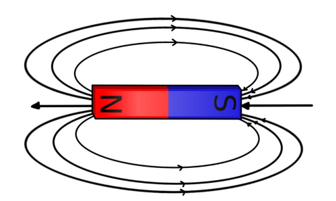2. List the properties of magnetic field lines.

Magnetic field lines possess the following properties:

• They are do not intersect with each other.
• They emerge from north pole and merge at south pole.
• The direction of field lines is from south pole to north pole.

3. Why don’t two magnetic field lines intersect each other?

If two magnetic field lines intersect, they will start pointing to different directions. Any compass placed at this point of intersection will have to show two directions. This is not possible. Hence, two magnetic lines never intersect.

### NCERT Solutions for Class 10 Science Chapter 13 – Intext Questions – Page 229

1. Consider a circular loop of wire lying in the plane of the table. Let the current pass through the loop clockwise. Apply the right-hand rule to find out the direction of the magnetic field inside and outside the loop.

From the above diagram we can see that for the downward direction of the current, the direction of the magnetic field will be as if emerging from the table outside the loop and merging the table inside the loop. Similarly, for upward direction of current, the direction of the magnetic field will be as if they are emerging from the table outside the loop and merging into the table inside the loop.

2. The magnetic field in a given region is uniform. Draw a diagram to represent it.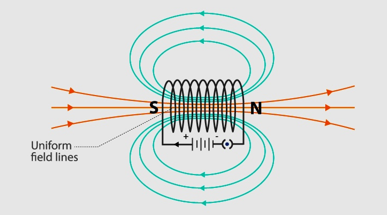3.Choose the correct option.

The magnetic field inside a long straight solenoid-carrying current

(a) is zero.

(b) decreases as we move towards its end.

(c) increases as we move towards its end.

(d) is the same at all points.

(d) Is the same at all points; uniform magnetic field throughout

### Intext Questions – Page 231

1. Which of the following property of a proton can change while it moves freely in a magnetic field? (There may be more than one correct answer.)

(a) mass
(b) speed
(c) velocity
(d) momentum

(c ) velocity and (d) momentum ; due to force applied by magnetic field the proton changes its path into a circular one.

2. In Activity 13.7, how do we think the displacement of rod AB will be affected if (i) current in rod AB is increased; (ii) a stronger horse-shoe magnet is used; and (iii) length of the rod AB is increased?

Any current carrying conductor when placed in a magnetic field experiences force. This experienced force depends on the amount of current, strength of magnetic field, and the length of a conductor. Hence,

• Displacement of rod AB is increased with increase in current in rod AB.
• Displacement of rod AB is increased if a stronger horse-shoe magnet is used.
• Displacement of rod AB is increased with increase in length of rod AB.

3. A positively-charged particle (alpha-particle) projected towards west is deflected towards north by a magnetic field. The direction of magnetic field is

(a) towards south
(b) towards east
(c) downward
(d) upward

(d) Upward; we can find this by using Fleming’s left hand rule.

### Intext Questions – Page 233

1. State Fleming’s left-hand rule.

Fleming’s Left hand rule says that if we arrange our thumb, forefinger and middle finger of the left hand such that they are at right angles to each other, then the thumb points towards the direction of the magnetic force, the forefinger points towards the direction of magnetic field and the middle finger points towards the direction of the current.

2. What is the principle of an electric motor?

An electric motor works on the principle of magnetic effect of current. A current carrying loop experiences force and rotates when placed in a magnetic field. The direction of rotation can be determined by Fleming’s left hand rule.

3. What is the role of the split ring in an electric motor?

A split ring acts as a commutator in an electric motor. Its job is to reverse the direction of the current each time the half rotation of the coil is completed. This enables the coil to keep rotating in the same direction.

### NCERT Solutions for Class 10 Science Chapter 13 – Intext Questions – Page 236

1. Explain different ways to induce current in a coil.

We can induce current in a coil by moving the coil rapidly between the two poles of horse shoe magnet. Current is also induced in a coil when a magnet is moved relative to the coil.

### Intext Questions – Page 237

1. State the principle of an electric generator.

Electric generator operates on the principle of electromagnetic induction. A rotating coil when placed in a magnetic field, generates current.

2. Name some sources of direct current.

Some sources of direct current are:

(i) DC generator
(ii) Cell and Batteries

3. Which sources produce alternating current?

Alternating current is produced by:

(i) Power plants
(ii) AC generators
(iii) Hydro-electric plants

4. Choose the correct option.

A rectangular coil of copper wires is rotated in a magnetic field. The direction of the induced current changes once in each

(a) two revolutions
(b) one revolution
(c) half revolution
(d) one-fourth revolution

(c ) half revolution

### Intext Questions – Page 238

1. Name two safety measures commonly used in electric circuits and appliances.

Two safety measures commonly used in electric circuits and appliances are:

(i) Fuse: The fuse melts to stop the flow of excessive current into appliances to avoid any damage to them.

(ii) Earthing: Used to avoid any electric shocks. Any leakage of current in an appliance is transferred to ground by earthing saving the users from electric shocks.

2. An electric oven of 2 kW power rating is operated in a domestic electric circuit (220 V) that has a current rating of 5 A. What result do you expect? Explain.

We know, P = V x I

Where, P = power, V = potential difference, and I = current.

=> I = P / V

=> I = 2000 W / 220 V

=> I = 9.09 A

We see that the current drawn by the electric oven is more than the current rating of the domestic electric circuit. Hence, the fuse will melt and the circuit would be broken.

3. What precaution should be taken to avoid the overloading of domestic electric circuits?

(i) Usage of too many appliances at the same time should be avoided.

(ii) Too many appliances should not be connected to the same socket.

(iii) Fuse and earthing should be checked and maintained.

(iv) Faulty appliances and sockets must not be used.

### NCERT Solutions for Class 10 Science Chapter 13 – NCERT Exercise – Page 240

1. Which of the following correctly describes the magnetic field near a long straight wire?

a) The field consists of straight lines perpendicular to the wire.

b) The field consists of straight lines parallel to the wire.

c) The field consists of radial lines originating from the wire.

d) The field consists of concentric circles centered on the wire.

(d) The field consists of concentric circles centred on the wire.

2. The phenomenon of electromagnetic induction is

(a) the process of charging a body.

(b) the process of generating magnetic field due to a current passing through a coil.

(c) producing induced current in a coil due to relative motion between a magnet and the coil.

(d) the process of rotating a coil of an electric motor.

( c) producing induced current in a coil due to relative motion between a magnet and the coil.

3. The device used for producing electric current is called a

(a) generator

(b) galvanometer

(c) ammeter

(d) motor

(a) generator

4. The essential difference between an AC generator and a DC generator is that

(a) AC generator has an electromagnet while a DC generator has permanent magnet.

(b) DC generator will generate a higher voltage.

(c) AC generator will generate a higher voltage.

(d) AC generator has slip rings while the DC generator has a commutator.

(d) AC generator has slip rings while the DC generator has a commutator.

5. At the time of short circuit, the current in the circuit

(a) reduces substantially.

(b) does not change.

(c) increases heavily.

(d) vary continuously.

(c ) increases heavily

6. State whether the following statements are true or false.

(a) An electric motor converts mechanical energy into electrical energy.

(b) An electric generator works on the principle of electromagnetic induction.

(c) The field at the centre of a long circular coil carrying current will be parallel straight lines.

(d) A wire with a green insulation is usually the live wire of an electric supply.

(a) False; Converts electrical energy to mechanical energy.

(b) True

(c) True

(d) False; A wire with red insulation is usually the live wire.

7. List two methods of producing magnetic fields.

We can produce magnetic field by using current carrying conductors and bar magnets.

8. How does a solenoid behave like a magnet? Can you determine the north and south poles of a current–carrying solenoid with the help of a bar magnet? Explain.

A solenoid is formed when an insulated copper wire forms continuous circular loops. A magnetic field is produced around it when we pass current through it. This magnetic field is similar to the magnetic field produced by a bar magnet. We can see the arrangement in the shown figure.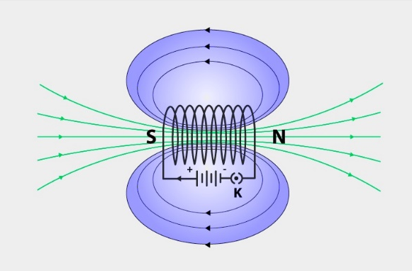We can determine the north and south poles with the help of a bar magnet by bringing the north pole of the magnet close to the negative terminal of the cell. We observe that the solenoid repels the magnet and as similar poles repel each other, we can infer that the end connected to the negative terminal acts as north pole and the other end is south pole.

9. When is the force experienced by a current–carrying conductor placed in a magnetic field largest?

When the direction of the current is perpendicular to the direction of magnetic field, the force experienced by the current carrying conductor is the maximum.

10. Imagine that you are sitting in a chamber with your back to one wall. An electron beam, moving horizontally from back wall towards the front wall, is deflected by a strong magnetic field to your right side. What is the direction of magnetic field?

We can determine the direction of the magnetic field by using Fleming’s left hand rule. Magnetic field will be perpendicular to the direction of the current inside the chamber. The direction of the current is from the front wall to the back wall as negatively charged electrons are moving from the back wall to the front wall. Given, the direction of magnetic force is rightward. This gives us the result that the direction of the magnetic field inside the chamber is downward.

11. Draw a labelled diagram of an electric motor. Explain its principle and working. What is the function of a split ring in an electric motor?

Electric motor is used to convert electrical energy into mechanical energy. It works on the principle of magnetic effect of current.

An electric motor consists of a rectangular coil ABCD of insulated copper wire. The coil is placed between the two poles of a magnetic field such that the arm AB and CD are perpendicular to the direction of the magnetic field. The ends of the coil are connected to the two halves P and Q of a split ring. The inner sides of these halves are insulated and attached to an axle. The external conducting edges of P and Q touch two conducting stationary brushes X and Y, respectively.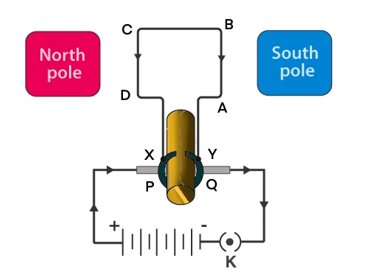When current is made to flow through the coil ABCD by closing the switch, the coil starts to rotate in the anticlockwise direction. This is due to the downward force acting on the length AB and simultaneously an upward force acting along the length CD. As a result of which the coil rotates in the anticlockwise direction. Current in the length AB flows from A to B and the magnetic fields act from left to right normal to the length AB. According to Fleming’s Left Hand rule, a downward force acts along the length AB. Similarly, the current along the length CD flows from C to D and the magnetic field acts from left to right. Therefore, an upward force acts along the length CD. These two forces together cause the coil to rotate anti-clockwise. After half a rotation, the position of AB and CD interchange. The half ring P come in contact with brush X and the half ring Q comes in contact with brush Y. Hence the direction of current in the coil ABCD gets reversed.

12. Name some devices in which electric motors are used.

Electric motors are used in electric fans, water pumps, washing machines, mixers, etc.

13. A coil of insulated copper wire is connected to a galvanometer. What will happen if a bar magnet is (i) pushed into the coil, (ii) withdrawn from inside the coil, (iii) held stationary inside the coil?

(i) When a bar magnet is pushed into the coil, current is induced in the coil for an instant and the galvanometer deflects in a particular direction momentarily.

(ii) When the bar magnet is withdrawn from inside the coil, current is induced  in the opposite direction for a moment and the galvanometer deflects in the opposite direction for an instant.

(iii) When the bar magnet is held stationary inside the coil, no current will be induced and there will be no deflection in the galvanometer.

14. Two circular coils A and B are placed closed to each other. If the current in the coil A is changed, will some current be induced in the coil B? Give reason.

Yes, some current will be induced in coil B because when the current in coil A changes, the magnetic field associated with it also changes. Since they are in close proximity, magnetic field around coil B also undergoes change. The change in the magnetic field of coil B induces current in it. .

15. State the rule to determine the direction of a (i) magnetic field produced around a straight conductor-carrying current, (ii) force experienced by a current-carrying straight conductor placed in a magnetic field which is perpendicular to it, and (iii) current induced in a coil due to its rotation in a magnetic field.

(i) Maxwell’s right hand thumb rule

(ii) Fleming’s left hand rule

(iii) Fleming’s right hand rule

16. Explain the underlying principle and working of an electric generator by drawing a labelled diagram. What is the function of brushes?

Given is the diagram of a electric generator.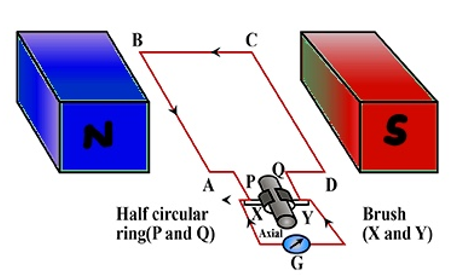In the given figure, X and Y are brushes, P and Q are slip rings. We also have an axle and a galvanometer.

When the axle is rotated clockwise, AB moves upwards and CD moves downwards. The movement of AB and CD in the magnetic field results in the production of electric current due to electromagnetic induction. AB moves upwards and the magnetic fields act from left to right. Therefore, according to Fleming’s right hand rule, the direction of the induced current will be from A to B along the length AB. Similarly, the direction of the induced current will be from C to D along the length CD. The direction of the current in the coil is ABCD. Hence, galvanometer shows a deflection in a particular direction.

After half a rotation, length AB starts moving downwards while the length CD starts moving upwards. Now, the direction of the induced current reverses to DCBA. And this process keeps repeating. As the direction of the induced current reverses every half rotation, the current induced is known as alternating current.

Brushes play an important role of transferring current from coil to the external circuit.

17. When does an electric short circuit occur?

Short circuit occurs when:

1. Socket or power supply is overloaded.
2. When live wires whose insulation have worn off come in contact with each other.

18. What is the function of an earth wire? Why is it necessary to earth metallic appliances?